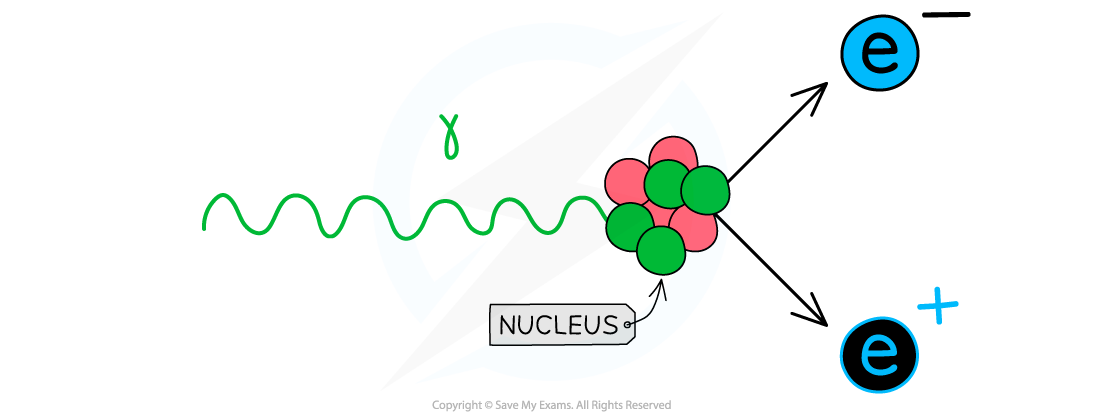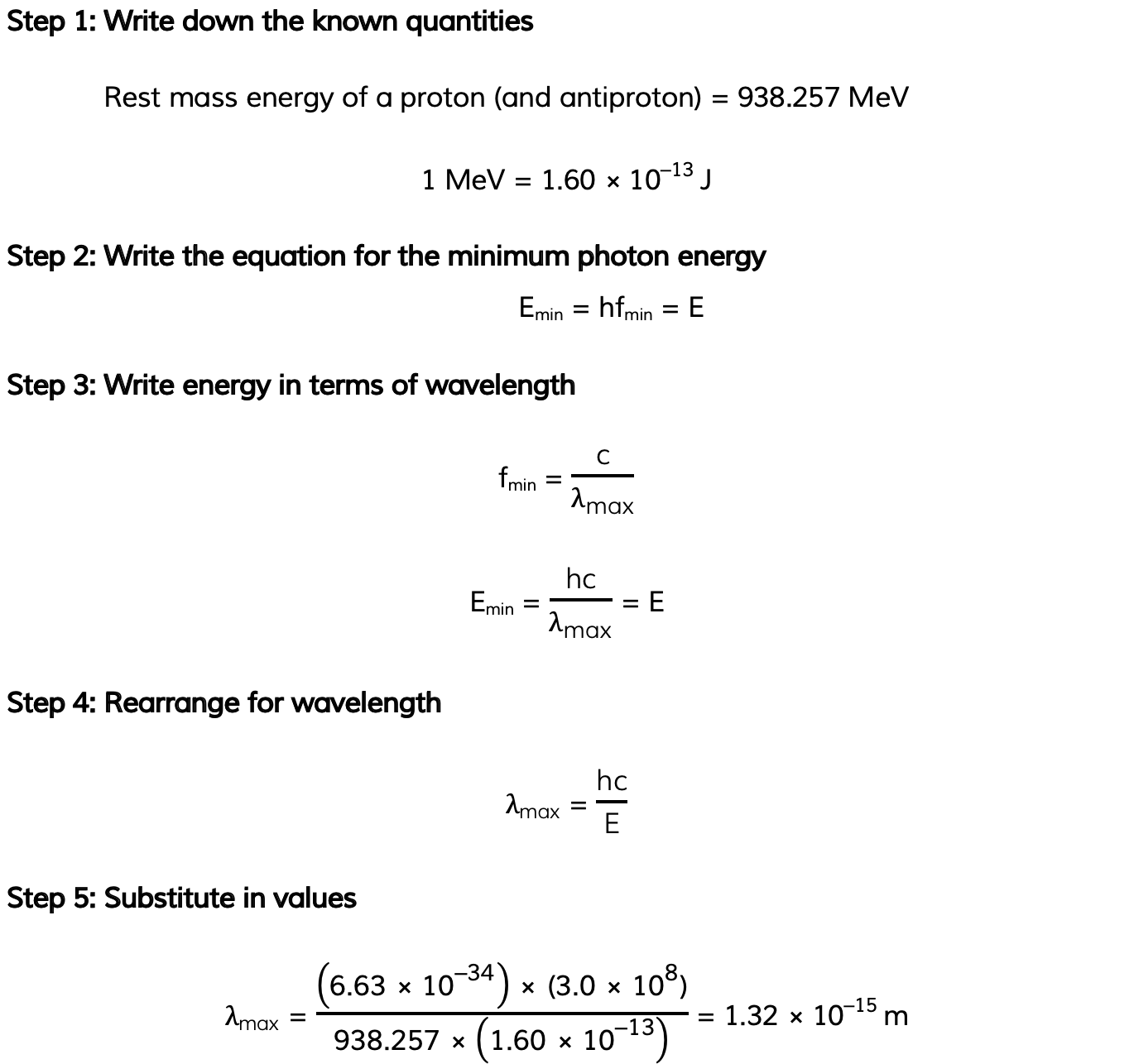# IB DP Physics: HL复习笔记12.1.6 Pair Production & Annihilation

### Pair Production & Annihilation

#### Annihilation

• When a particle meets its antiparticle pair, the two will annihilate
• Annihilation is:

When a particle meets its equivalent anti–particle they both are destroyed and their mass is converted into energy in the form of two gamma ray photonsWhen an electron and positron collide, their mass is converted into energy in the form of two photons emitted in opposite directions

• The minimum energy of one photon after annihilation is the total rest mass energy of one of the particles is:

Emin = hfmin = E

• Where:
• Emin = minimum energy of one of the photons produced (J)
• h = Planck's Constant (J s)
• fmin = minimum frequency of one of the photons produced (Hz)
• E = rest mass energy of one of the particles (J)
• To conserve momentum, the two photons will move apart in opposite directions
• To conserve energy, the two photons will have the same energy, which means they also have the same frequency because

E = hf

#### Pair Production

• Pair production is the opposite of annihilation
• Pair production is:

When a photon interacts with a nucleus or atom and the energy of the photon is used to create a particle–antiparticle pair

• The presence of a nearby neutron is essential in pair production so that the process conserves both energy and momentum
• A single photon alone cannot produce a particle–anti-particle pair or the conservation laws would be broken
• Pair creation is a case of energy being converted into matterWhen a photon with enough energy interacts with a nucleus it can produce an electron-positron pair

• This means the energy of the photon must be above a certain value to provide the total rest mass energy of the particle–antiparticle pair
• The minimum energy for a photon to undergo pair production is the total rest mass energy of the particles produced:

Emin = hfmin = 2E

• Where:
• Emin = minimum energy of the incident photon (J)
• h = Planck's Constant (J s)
• fmin = minimum frequency of the photon (Hz)
• E = rest mass energy of one of the particles (J)
• To conserve momentum, the particle and anti–particle pair move apart in opposite directions
• Remember for both calculations, the frequency f is also defined by the wave equation, v = fλ
• Using this equation, the wavelength λ can also be calculated
• Remember that v is c (the speed of light) for gamma ray photons

#### Worked Example

Calculate the maximum wavelength of one of the photons produced when a proton and antiproton annihilate each other.#### Exam Tip

Since the Planck constant is in Joules (J) remember to always convert the rest mass-energy from MeV to J.